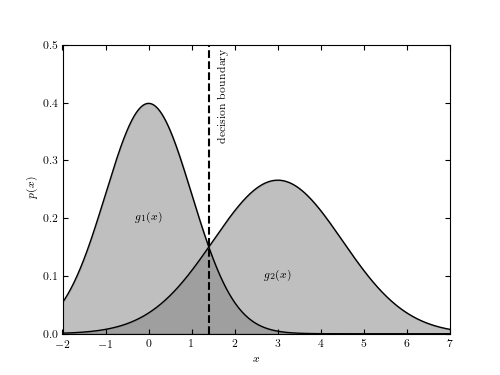# Bayes Decision Boundary¶

Figure 9.1

An illustration of a decision boundary between two Gaussian distributions.```
```
```# Author: Jake VanderPlas
#   The figure produced by this code is published in the textbook
#   "Statistics, Data Mining, and Machine Learning in Astronomy" (2013)
#   To report a bug or issue, use the following forum:
import numpy as np
from matplotlib import pyplot as plt
from scipy.stats import norm

#----------------------------------------------------------------------
# This function adjusts matplotlib settings for a uniform feel in the textbook.
# Note that with usetex=True, fonts are rendered with LaTeX.  This may
# result in an error if LaTeX is not installed on your system.  In that case,
# you can set usetex to False.
if "setup_text_plots" not in globals():
from astroML.plotting import setup_text_plots
setup_text_plots(fontsize=8, usetex=True)

#------------------------------------------------------------
# Compute the two PDFs
x = np.linspace(-3, 7, 1000)
pdf1 = norm(0, 1).pdf(x)
pdf2 = norm(3, 1.5).pdf(x)
x_bound = x[np.where(pdf1 < pdf2)]

#------------------------------------------------------------
# Plot the pdfs and decision boundary
fig = plt.figure(figsize=(5, 3.75))
ax.plot(x, pdf1, '-k', lw=1)
ax.fill_between(x, pdf1, color='gray', alpha=0.5)

ax.plot(x, pdf2, '-k', lw=1)
ax.fill_between(x, pdf2, color='gray', alpha=0.5)

# plot decision boundary
ax.plot([x_bound, x_bound], [0, 0.5], '--k')

ax.text(x_bound + 0.2, 0.49, "decision boundary",
ha='left', va='top', rotation=90)

ax.text(0, 0.2, '\$g_1(x)\$', ha='center', va='center')

ax.text(3, 0.1, '\$g_2(x)\$', ha='center', va='center')

ax.set_xlim(-2, 7)
ax.set_ylim(0, 0.5)

ax.set_xlabel('\$x\$')
ax.set_ylabel('\$p(x)\$')

plt.show()
```